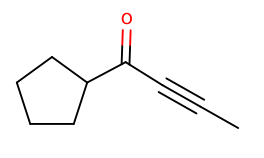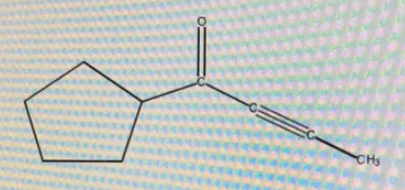# Problem: How many degrees of unsaturation does this compound have?

###### FREE Expert Solution

We’re being asked to determine the degrees of unsaturation for the given structure:Recall that the degrees of unsaturation or index of hydrogen deficiency (IHD) tell us how many hydrogens are missing in a structure.

In a given structure, the IHD is:

Rings: +1

Double bonds: +1

Triple bonds: +2

For a given structure, we simply need to determine the number of rings, double and triple bonds to determine the IHD.

80% (205 ratings)###### Problem Details

How many degrees of unsaturation does this compound have?